# SQL Aggregate Statement

## Introduction

In this chapter, we will learn how to use a SQL Aggregate statement with various options.

## SQL Aggregate Function

The Aggregate function is used to perform calculations on a set of values and return a single value, ignoring all null values.

The Aggregate functions are often used with the group by clause of the select statement in SQL

The database management aggregate function is a function in which the values of multiple rows are grouped together as input on certain criteria to form a single value of more significant meaning

All aggregate statements are deterministic In other words, aggregate functions return the same value each time that they are called when called with a specific set of input values.

See Deterministic and Nondeterministic Functions for more information about function determinism The over clause may follow all aggregate functions, except the STRING_AGG, grouping, or GROUPING_ID functions

Aggregate functions can we use the expressions only in the following situations
• The select list of a select statement (either a subquery or an outer query).
• A having clause.

## Functions

SQL provides the following aggregate functions based off of the following Student table

Syntax
1. SELECT * FROM Student;
Example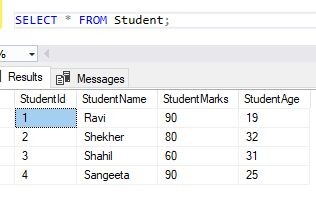## APPROX_COUNT_DISTINCT()

This statement returns the approximate number of unique non-null values in a group.

Approx_count_distinct expression evaluates an expression for each row in a group and returns the approximate number of unique non-null values in a group.

This function is designed to provide aggregations across large data sets where responsiveness is more critical than absolute precision.

Approx_count_distinct is designed for use in big data scenarios and is optimized for the following conditions
• Access of data sets that are millions of rows or higher and
• Aggregation of a column or columns that have many distinct values
This function implementation guarantees up to a 2% error rate within a 97% probability.Approx_count_distinct requires less memory than an exhaustive count distinct operation, given the smaller memory footprint

Approx_count_distinct is less likely to spill memory to disk compared to a precise count distinct operation.

Syntax
1. SELECT Count (StudentId) AS Approx_Distinct_OrderKey
2. FROM Student;
Example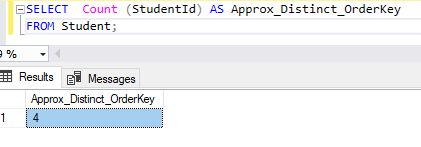## AVG ()

The AVG>statement calculates the average of non-null values in a set.

The AVG is the data type of expression is an alias data type, the return type is also of the alias data type.

However, if the base data type of the alias data type is promoted, for example from tinyint to int, the return value will take the promoted data type, and not the alias data type

AVG () computes the average of a set of values by dividing the sum ofthose values by the count of non-null values

If the sum exceeds the maximum value for the data type of the return value, AVG() will return an error. AVG is a deterministic function when used without the over and orderby clauses. It is non-deterministic when specified with the over and order by clauses. for more information.

Syntax
1. SELECT AVG(StudentAge) FROM Student;
Example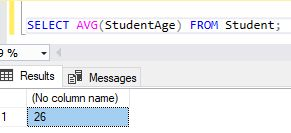## CHECKSUM_AGG()

This statement returns the checksum of the values in a group. CHECKSUM_AGG ignores null values.

The over clause can follow CHECKSUM_AGG

CHECKSUM_AGG can detect changes in a table

The CHECKSUM_AGG result does not depend on the order of the rows in the table. Also, CHECKSUM_AGG functions allow the use of the Distinct keyword and the Group By clause

If an expression list value changes, the list checksum value list will also probably change. However, a small possibility exists that the calculated checksum will not change

Syntax
1. SELECT CHECKSUM_AGG(CAST(StudentID AS int))
2. FROM Student;
3. GO
Example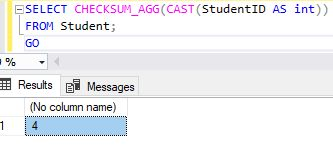## COUNT()

It is used to count the number of rows returned in a select statement.

Count function returns the number of items found in a group. count  operates like the COUNT_BIG function

These functions differ only in the data types of their return values.

Count always returns an int data type value.COUNT_BIG always returns a bigint data type value.

Syntax
1. SELECT COUNT (StudentName) from Student
Example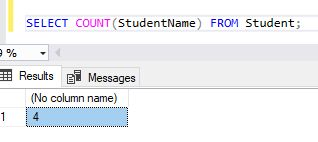## COUNT_BIG ()

This statement returns the number of items found in a group.COUNT_BIG operates like the count function. These functions differ only in the data types of their return values.

COUNT_BIG always returns a bigint data type value. Count always returns an int data type value.

The COUNT_BIG(*) returns the number of items in a group. This includes null values and duplicates.

The  COUNT_BIG (all expression) evaluates the expression for each row in a group and returns the number of nonnull values.

COUNT_BIG (distinct expression) evaluates the expression for each row in a group and returns the number of unique, nonnull values.

Syntax
1. SELECT COUNT(*)
2. FROM Student
3. GO
Example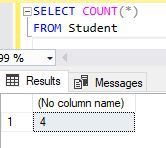## GROUPING ()

The Grouping statement indicates whether a specified column expression in a Group by  list is aggregated or not Grouping returns 1 for aggregated or 0 for not

Aggregated in the result set. Grouping can be used only in the SELECT <select> list, HAVING, and ORDER BY clauses when group by is specified.

Grouping is used to distinguish the null values that are returned by Rollup, cube, or Grouping sets from standard null values.

The null returned as the result of a Rollup, Cube, or grouping sets operation is a special use of null. This acts as a column placeholder in the result set and means all.

Syntax
1. SELECT GROUPING(StudentName) AS 'Grouping'
2. FROM Student
3. GROUP BY StudentName  WITH ROLLUP;
4. GO
Example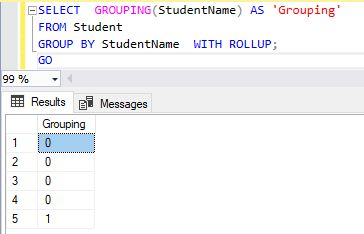## GROUPING_ID statement ()

This function computes the level of grouping. GROUPING_ID can be used only in the SELECT <select> list, HAVING, or ORDER BY clauses when GROUP BY is specified.

Syntax
1. SELECT GROUPING_ID(StudentId, StudentName)
2. FROM Student
3. GROUP BY CUBE(StudentId, StudentName)
Example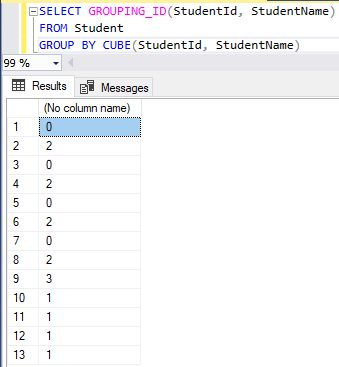## Summary

In this chapter, we learned how to use a SQL CAST and CONVERT statement with various options.
Next » SQL DATE TIME Statement